Fluid Mechanics
Volume 1, Issue 1, July 2015, Pages: 1-4

Numerical Simulation of Vortex Shedding Past a Single Cylinder Confined in a Channel

Dhananjay Yadav1, *, Ritesh Srivastava2, Jinho Lee1

1School of Mechanical Engineering, Yonsei University, Seoul, South Korea

2Department of Mechanical and Automation Engineering, Amity University, Noida, UP, India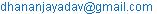(D. Yadav)

Dhananjay Yadav, Ritesh Srivastava, Jinho Lee. Numerical Simulation of Vortex Shedding Past a Single Cylinder Confined in a Channel. Fluid Mechanics. Vol. 1, No. 1, 2015, pp. 1-4. doi: 10.11648/j.fm.20150101.11

Abstract: The vortex shedding past a circular cylinder in a two- dimensional channel of varying height is presented in the term of Strouhal number by solving continuity and momentum equations using FLUENT 6.3. The computational grid structure is generated by using Gambit. In this analysis, the result is carried out with blockage ratio b=80, 0.83, 0.85, 0.88 and Reynolds number range from 50 to 300.

Keywords: Strouhal Number, Vortex Shedding, Blockage Ratio

1. Introduction

The cross flow close to a solid surface is of considerable interest due to their numerous applications in different field including the flow over heat exchanger tubes, seabed pipelines and experimental models in wind tunnel. Efforts have been made by several researchers to understand the effects of solid wall on the flow past a circular cylinder. The experimental studies carried out by Bearman and Zdravkovich , Buresti and Lanciotti , Angrilli et al. , Zdravkovich  and Taniguchi and Miyakoshi . Broadly speaking on these studies, they have shown that the vortex shedding is completely suppressed when the cylinder is closer than a critical distance from the wall.

However, almost all the experimental studies are at reasonably higher Reynolds numbers in the range on O(104)–O(105) where turbulence and three-dimensionality are important. Studies at low Reynolds numbers are relatively scarce. The experiential studied to estimate the effects of tunnel blockage on the span wise correlation of the wake of a circular cylinder over a range of Reynolds numbers that varied between 2400 and 4000 was carried out by Blackburn . It was observed that blockage increases the span wise correlation of the wake. Chen et al.  gave the numerically simulated a centrally placed cylinder with a focus on the nature and occurrence of the bifurcation from steady symmetric flow to the periodic shedding regime. The effects of a closely placed solid wall was not given due attention. The effect of varying gap between the cylinder and the channel walls was analysed by Zovatto and Pedrizzetti . Sahin and Owens  have presented a parameter for study the transition from steady two-dimensional flow to periodic vortex shedding across a range of blockage ratio. They found that the critical Reynolds number for such a transition increased with increasing blockage ratio. At increased blockage ratios, the flow alters significantly with vortices beginning to be shed from both the cylinder and the walls. Rehimi et al.,  have observed in both two- and three-dimensional studies at a given distance downstream of the cylinder depending on the blockage ratio and the Reynolds number. As for the transition from two-dimensional to three-dimensional flow for a single blockage ratio of 0.20 was studied by Camarri and Gianetti  using linear stability analysis and obtained that the inversion had little effect on the types of mode of transition and that the critical Reynolds numbers. Griffith et al.  have studied the vortex shedding and three-dimensional behaviour of flow past a cylinder confined in a channel with blockage ration 0.2, 0.33 and 0.5 using spectral-element solver and observed that Strouhal number increases with blockage ratio 0.2, 0.3 and 0.5.

In the present paper, the simulation is carried out with blockage ratio 0.80, 0.83, 0.85, 0.88 and 0.9 using FLUENT 6.3 solver. It is observed that Strouhal number decreases up to blockage ratio 0.83 to 0.9. It is differ for the blockage ratio 0.2, 0.3 and 0.5 as was studied by Griffith et al. . This happens because for the blockage ratio greater 0.83, the velocity becomes very high and pressure becomes very low. To the best of our knowledge, there is no work with these blockage ratios. To validate the solution, the results are also obtained for the blockage ratio 0.2 and compared with results obtained by Griffith et al. .

2. Problem Statement for Single Circular Cylinder

Consider the 2-D flow of air across an infinitely long circular cylinder confined in a channel as shown in Fig. 1. The length and the height of the computational domain are defined in terms of the axial and lateral dimensions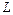and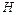, respectively.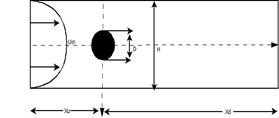Fig. 1. Sketch of the geometry.

The cylinder is placed at upstream and downstream distances of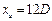and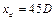, respectively and the total length of the computational domain is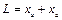in the axial direction, where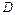is the diameter of the cylinder.

3. Governing Equations and Boundary Conditions

The equations governing incompressible viscous fluid flow in two-dimensions are the continuity equation and the two components of momentum equation. In absence of body forces and heat transfer, these equations can be expressed in the conservative non dimensional primitive variable form as follows :

Continuity Equation: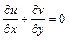(1)

X-Momentum equation: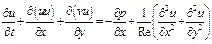(2)

Y-Momentum equation: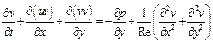(3)

The following boundary conditions in their dimensionless forms are used.

The inlet boundary conditions are given by a prescribed parabolic profile,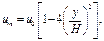where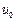and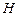are the centre line velocity and the distance between the side walls, respectively.

On the circular obstacle: No slip condition is used i.e.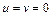.

At upper and lower boundaries: No-slip condition of velocity is used i.e..

At the exit boundary: The Neumann boundary condition as: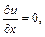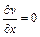The simulation studies are carried out for several values of Reynolds numbers (Re), based on the centre line in flow velocity and diameter (D) of the cylinder, and normalized channel height (D/H). The considered values lie in the ranges Reynolds number from 50 to 300 and blockage ratio b=0.83 0.85, 0.88. To validate the solution, we also considered the blockage ratio b=0.2. The parameter space considered in the study is shown in Table 1.

Table 1. Reynolds numbers and channel heights considered for single circular cylinder.

 Reynolds number (Re) 50, 100 to 300 in steps of 50 Blockage ratio b (=D/H) 0.2, 0.83, 0.85 and 0.88.

4. Numerical Methodology and Results

The problem under consideration is solved by commercial software package FLUENT (6.3). The grid is generated by using GAMBIT. The second order upwind scheme is used to discretise convective terms of momentum equations while the diffusive term is discretised by central difference scheme. To validate the solution, calculations is carried out first for the blockage ratio b=0.2. The Strouhal number for different values of Reynolds numbers at blockage ratio 0.2 is plotted in Fig. 2 and compared our results with the results given in Griffith et al. . From these, we noted that the agreement is very good and this verifies the results obtained in the present work. The Reynolds number is defined as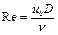, whereis the diameter of the circular cylinder and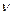is the kinematic viscosity. The Strouhal number is defined as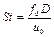, where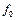is the frequency of vortex shedding. The blockage ratio is defined as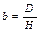, where H is the width of the channel.

Flow behaviour past single cylinders confined in a channel with different blockage ratio has been studied. Simulation has been carried out for the blockage ratio b=0.83, 0.85 and 0.88. Fig.3 shows the plot of Strouhal number with Reynolds number for different values of the block ratio and tabulated in Table 2. It is observed that the Strouhal number increases with Reynolds number and decreases with blockage ratio 0.8 to 0.9. This happens because for the blockage ratio greater 0.85, the velocity becomes very high and pressure becomes very low.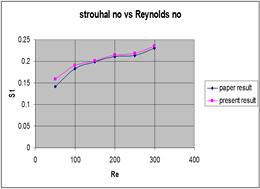Fig. 2. Variation of Strouhal number with Reynolds number for blockage ratio b=0.2 validation with the result of Griffith et al. .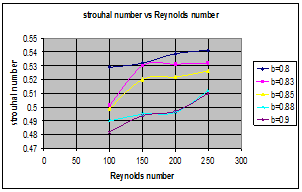Fig. 3. The variation of Strouhal number with Reynolds number for different values of blockage ratio.

Table 2. Comparison of Strouhal number with Reynolds number for different values of blockage ratio.

 Strouhal number at Strouhal number at Strouhal number at Strouhal number at Strouhal number at 100 0.531 0.501 0.499 0.49 0.482 150 0.534 0.530 0.520 0.495 0.494 200 0.539 0.531 0.522 0.496 0.497 250 0.542 0.532 0.526 0.512 0.510

Fig. 4 depicts plots of vorticity for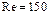and two blockage ratios,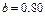(a) and(b). For the smallest blockage shown,, the wake bears similarity to the wake behind an unconfined cylinder. Vorticity is pulled from the walls, but only a small amount when compared to the other case. For blockage ratio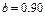, vortices forming on the walls of the channel can be seen being entrained into the vortex street.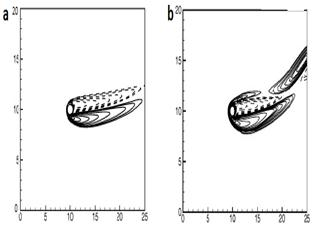Fig. 4. Vorticity plots for two blockage ratios,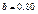(a) and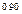(b) at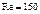.

The apparent in the two cases is the inversion of the vortex street; vortices forming on one side of the cylinder finish further downstream on the opposite channel. Also, it can be clearly seen that at low blockage ratios wall boundary layer has strong influence over around the cylinder so that it inhibits vortex shedding. As the blockage ratio increases computation, time required for developing unsteadiness increases.

Fig. 5 shows the streamline patterns at a certain instant forand two blockage ratios,(a) and(b). From the streamline patterns, it is seen that the flow pattern changes at different blockage ratios.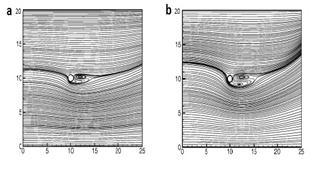Fig. 5. Streamline patterns for two blockage ratios,(a) and(b) at.

5. Conclusion

Motivated by the vortex shedding past a circular cylinder which is very useful in many practical applications including the flow over heat exchanger tubes, seabed pipelines and experimental models in wind tunnel in the present paper the effect of blockers ratio 0.80 to 0.9 on the Strouhal number was investigated. The results show that the Strouhal number decreases with increase in block ratio greater than 0.8. This illustrates that for these block ration vortex shedding frequency increases and coefficient of lift oscillates.

References

1. P. W. Bearman, M. M. Zdravkovich, "Flow around a circular cylinder near a plane boundary", Journal of Fluid Mechanics, vol. 89, 1978, pp. 33-47.
2. G. Buresti, A. Lanciotti, "Mean and fluctuating forces on a circular cylinder in cross-flow near a plane surface", J. of Wind Engng. Ind. Aerodyn., vol. 41, 1992, pp. 639-650.
3. F. Angrilli, G. Di Silvio, and A. Zanardo, "Hydroelasticity study of a circular cylinder in a water stream," In Flow Induced Structural Vibrations (ed. E. Naudascher), IUTAM-IAHR Symposium, 1974, pp. 504-512.
4. M. M. Zdravkovich, "Interference between two circular cylinders forming a cross", Journal of Fluid Mechanics, vol. 128, 1983, pp. 231-246.
5. S. Taniguchi, K. Miyakoshi, "Fluctuating fluid forces acting on a circular cylinder and interference with a plane wall" Experiments in Fluids , vol. 9, 1990, pp. 197-204.
6. M. H. Blackburn, "Effect of blockage on spanwise correlation in a circular cylinder wake", Experiment in Fluids, vol. 18, 1994, pp. 134–136.
7. J. H. Chen, W. G. Pritchard, S. J. Tavener, "Bifurcation of flow past a cylinder between parallel plates", Journal of Fluid Mechanics, vol. 284, 1995, pp. 23–41.
8. L. Zovatto, G. Pedrizzetti, "Flow about a circular cylinder between parallel walls", Journal of Fluid Mechanics, vol. 440, 2001, pp. 1–25.
9. M. Sahin, R. G. Owens, "A numerical investigation of wall effects up to high blockage ratios on two-dimensional flow past a confined circular cylinder", Physics of Fluids, vol. 16, 2004, pp. 1305–1320.
10. F. Rehimi, F. Aloui, S. B. Nasrallah, L. Doubliez, J. Legrand, "Experimental investigation of a confined flow downstream of a circular cylinder centred between two parallel walls", Journal of Fluids and Structures, vol. 24, 2008, pp. 855–882.
11. S. Camarri, F. Gianetti, "Effect of confinement on three-dimensional stability in the wake of a circular cylinder", Journal of Fluid Mechanics, vol. 642, 2010, pp. 477–487.
12. M. D. Griffith, J. Leontini, M. C.Thompson, K. Hourigan, "Vortex shedding and three-dimensional behaviour of flow past a cylinder confined in a channel", Journal of Fluids and Structures, vol. 27, 2011, pp. 855–860.
13. P. Patil, S. Tiwari, "Three-dimensional numerical investigations on flow and heat transfer for flow past a channel confined square cylinder", Progress in Computational Fluid Dynamics, vol. 10, 2010, pp. 146-156.

 Contents 1. 2. 3. 4. 5.
Article ToolsAbstractPDF(256K)